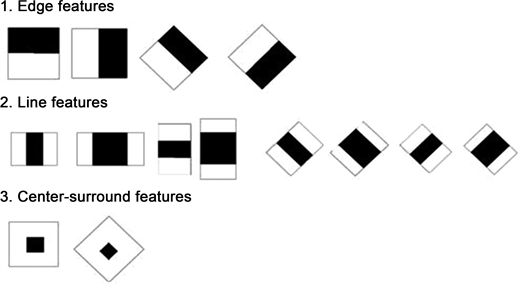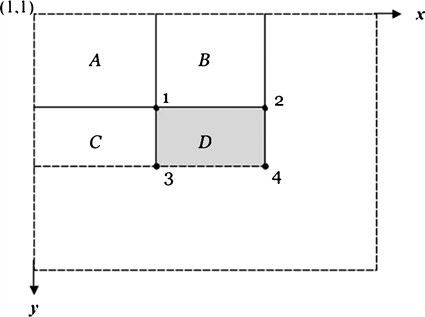# 复杂环境下的人脸识别增强算法Face Recognition Algorithm in Complex Environment of Mobile Platform

DOI: 10.12677/CSA.2018.811184, PDF, HTML, XML, 下载: 755  浏览: 1,492

Abstract: This paper studies the problem of slow and low accuracy of face recognition in complex environment and proposes a face recognition algorithm. Firstly, Adaboost combined with skin color segmentation for face detection. The advantages of fast skin color segmentation technique are used to screen out candidate face areas. Meanwhile, Haar features are used to train classifiers to further enhance detection performance and improve accuracy in complex background. Then, the face recognition method (LDA) based on linear discriminant analysis is adopted. LDA algorithm is a supervised learning method to classify samples. By applying the label information of samples, the optimal projection subspace is found to complete the classification. Experimental results show that the algorithm improves the recognition speed and accuracy greatly.

1. 引言

2. 人脸检测

${p}_{ij}={\sum }_{i\le x,j\le y}I\left(i,j\right)$ (1)Figure 1. haar characteristic diagramFigure 2. Value diagram of integral graph

1) 定义初始样本集 $\left({x}_{1},{y}_{1}\right),\left({x}_{2},{y}_{2}\right),\cdots ,\left({x}_{n},{y}_{n}\right)$ ，其中 ${y}_{i}$ 表示样本集中第i个样本的类别， ${y}_{i}=0$ 表示这个样本为非人脸样本， ${y}_{i}=1$ 表示这个样本为人脸样本。n为总的样本数量。

2) 初始各样本的权值分布：对于非人脸样本：

 (2)

m为非人脸样本个数。

${D}_{t}\left(i\right)=\frac{1}{2l}$ (3)

i为人脸样本个数， ${D}_{t}\left(i\right)$ 为第t次循环中第i个样本的误差权值。

3) 对于 $t=1,\cdots ,T$

${q}_{t,i}=\frac{{w}_{t,i}}{{\sum }_{j=1}^{n}{w}_{t,j}}$ (4)

${\epsilon }_{f}={\Sigma }_{i}{y}_{i}\cdot |h\left({x}_{i},f,P,\theta \right)-{y}_{i}|$ (5)

4) 最后的强分类器为：

$C\left(x\right)=\left\{\begin{array}{l}1,\text{\hspace{0.17em}}\text{\hspace{0.17em}}\text{\hspace{0.17em}}\text{\hspace{0.17em}}{\sum }_{t=1}^{T}{a}_{t}{h}_{t}\left(x\right)\ge \frac{1}{2}{\sum }_{t=1}^{T}{a}_{t}\\ 0,\text{\hspace{0.17em}}\text{\hspace{0.17em}}\text{\hspace{0.17em}}\text{\hspace{0.17em}}\text{\hspace{0.17em}}\text{\hspace{0.17em}}\text{\hspace{0.17em}}\text{\hspace{0.17em}}\text{\hspace{0.17em}}\text{\hspace{0.17em}}\text{\hspace{0.17em}}\text{\hspace{0.17em}}其它\end{array}$ (6)

2.2. 肤色模型

$190<{c}_{b}+0.6{c}_{r}<215$ (7)

2.3. 算法效果分析

1) 利用肤色分割算法确定人脸的大致范围。Table 1. Compares the three algorithms

3. 人脸识别

3.1. 基于LDA的人脸识别算法

Fisher判别准则：

${m}_{i}=\frac{1}{{n}_{i}}{\sum }_{x\in {x}_{i}}x,\text{\hspace{0.17em}}\text{\hspace{0.17em}}i=1,2$ (8)

${S}_{i}={\sum }_{x\in X}\left(x-{m}_{i}\right){\left(x-{m}_{i}\right)}^{\text{T}}$ (9)

${S}_{b}=\left({m}_{1}-{m}_{2}\right){\left({m}_{1}-{m}_{2}\right)}^{\text{T}}$ (10)

${J}_{F}\left(w\right)=\frac{{\left(\stackrel{¯}{{m}_{1}}-\stackrel{¯}{{m}_{2}}\right)}^{2}}{{\stackrel{¯}{{s}_{1}}}^{2}+{\stackrel{¯}{{s}_{2}}}^{2}}$ (11)

${\left(\stackrel{¯}{{m}_{1}}-\stackrel{¯}{{m}_{2}}\right)}^{2}$ 代表两个类的类间距离，其中 $\stackrel{¯}{{m}_{1}},\stackrel{¯}{{m}_{2}}$ 分别是两类均值， ${\stackrel{¯}{{s}_{1}}}^{2}+{\stackrel{¯}{{s}_{2}}}^{2}$ 代表类内距离。因 ${y}_{i}={w}^{\text{T}}{x}_{i}$ ，则

$\stackrel{¯}{{m}_{i}}=\frac{1}{{n}_{i}}{\sum }_{x\in {X}_{i}}y={w}^{\text{T}}x={w}^{\text{T}}{m}_{i}$ (12)

${\left(\stackrel{¯}{{m}_{1}}-\stackrel{¯}{{m}_{2}}\right)}^{2}={w}^{\text{T}}{s}_{b}w$ (13)

${\stackrel{¯}{{s}_{1}}}^{2}+{\stackrel{¯}{{s}_{2}}}^{2}={w}^{\text{T}}{s}_{w}w$ (14)

${s}_{b}w\left({w}^{\text{T}}{s}_{w}w\right)-{s}_{w}w\left({w}^{\text{T}}{s}_{b}w\right)=0$ (15)

${s}_{b}w={J}_{F}\left(w\right){s}_{w}w$ (16)

${J}_{F}\left(w\right)=\lambda$ ，则，

${s}_{b}w=\lambda {s}_{w}w$ (17)

3.2. 人脸识别实验

1) 人脸数据库：样本数量为20张人脸，每个人脸2个样本。

2) 单人多次识别：在人脸姿态、表情、光照不同情况下，使被测人脸处在微低头、微抬头、微笑、皱眉、强光、弱光6种情况下下各随机进行5次测试，共30次。

3) 双人(在数据库中)同时多次识别：两人(A和B)随机采取不同站位、脸部姿态、表情时测试30次，统计结果。结果如表2所示。

4. 结论

NOTES

*通讯作者。

  王盾, 袁杰. 复杂环境下的人脸识别研究[J]. 自动化应用, 2017(11): 75-78. https://doi.org/10.3969/j.issn.1674-778X.2017.11.036  黄鑫材. 复杂环境下的人脸识别系统研究[D]: [硕士学位论文]. 杭州: 浙江工业大学, 2015.  何强, 文凯. 一种复杂背景下的人脸识别算法研究[J]. 电视技术, 2017(11): 207-213. https://doi.org/10.16280/j.videoe.2017.h11.040  沈继锋, 时士伟, 左欣, 等. 基于多通道图判别投影HAAR特征的多视角人脸检测[J]. 数据采集与处理, 2018(2): 270-279. https://doi.org/10.16337/j.1004-9037.2018.02.009  张薇, 王颖. 基于复杂背景下人脸图像识别相关算法的应用[J]. 电视技术, 2018(7): 20-24. https://doi.org/10.16280/j.videoe.2018.07.005  王冉. 基于Android平台人脸识别算法的应用[J]. 电子设计工程, 2017(16): 190-193. https://doi.org/10.3969/j.issn.1674-6236.2017.16.046  唐彪, 金炜, 符冉迪, 等. 多稀疏表示分类器决策融合的人脸识别[J]. 电信科学, 2018(4): 31-40. https://doi.org/10.11959/j.issn.1000-0801.2018010  白冰峰, 温秀兰, 张中辉. 基于Haar小波和形状模板的图像快速匹配算法[J]. 组合机床与自动化加工技术, 2017(2): 37-40. https://doi.org/10.13462/j.cnki.mmtamt.2017.02.010  糜元根, 陈丹驰, 季鹏. 基于几何特征与新Haar特征的人脸检测算法[J]. 传感器与微系统, 2017(2): 154-157. https://doi.org/10.13873/J.1000-9787(2017)02-0154-04  姜莹礁. 基于Gabor的人脸识别技术研究[J]. 海峡科技与产业, 2018(1): 66-67.  张贵英, 张先杰. 基于图像的人脸识别算法研究综述[J]. 电脑知识与技术, 2017(11): 165-167.